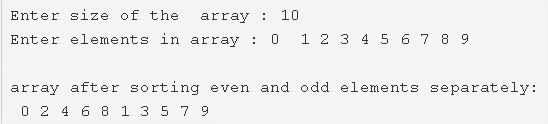# C Program To Sort Even And Odd Elements Of Array | C Programs

C program to sort even and odd elements of an array separately – In this article, we will discuss the numerous methods on how to sort even and odd elements of an array separately in C programming.

Suitable examples and sample programs have also been added so that you can understand the whole thing very clearly. The compiler has also been added with which you can execute it yourself.

The methods mentioned in this piece are as follows:

• Using Standard Method

As we all know, arrays are nothing but a basic sequential arrangement of a bunch of elements put together in any random fashion.Firstly, we need to enter the size of the array.

In this case, we can see that the size of the array entered is 10.

Later on, the elements of the array need to be entered.

The elements entered, in this case, are as follows:

0 1 2 3 4 5 6 7 8 9

Thus, once you separate sorting the even and odd elements, the array would look like this:

0 2 4 6 8 1 3 5 7 9

Thus, the methods used to sort even and odd elements in C programming are as follows:

## Using Standard Method

1. Read and store the array size into the variable n which is entered by the user.

2) Read and store the array elements in the array a[] using scanf statement and for loop and count the total number of odd numbers in the array.

3) Arrange the array elements in ascending order as follows.

a) For loop iterates from i=0 to i<n-1

b) The inner for loop iterates from j=0 to j<n-i-1

Compare the a[j] and a[j+1],if a[j] is highest element than a[j+1] then swap the both elements.Repeat this step until all iterations of j.

Repeat the above step until i<n-1.

4) Separate the even numbers and odd numbers of the arrays as follows

5) Initialize k=0 and j=n-c.

for loop iterates from i=0 to i<n

a) If a[i] is even number,if k<n-c then place the even number at b[k++].

b) If a[i] is odd number,if j<n then place the odd number at b[j++].

6) After 5th step, the array b[] contains even numbers from the index 0 to n-c-1 and odd numbers from n-c to n-1.

7) Move the array elements of b[] to the array a[] and print the array elements using for loop for(i=0;i<n;i++).

Output:
x

## C Program To Check If Alphabet, Digit or Special Character | C Programs

C program to check for an alphabet, digit or special character – In this article, ...